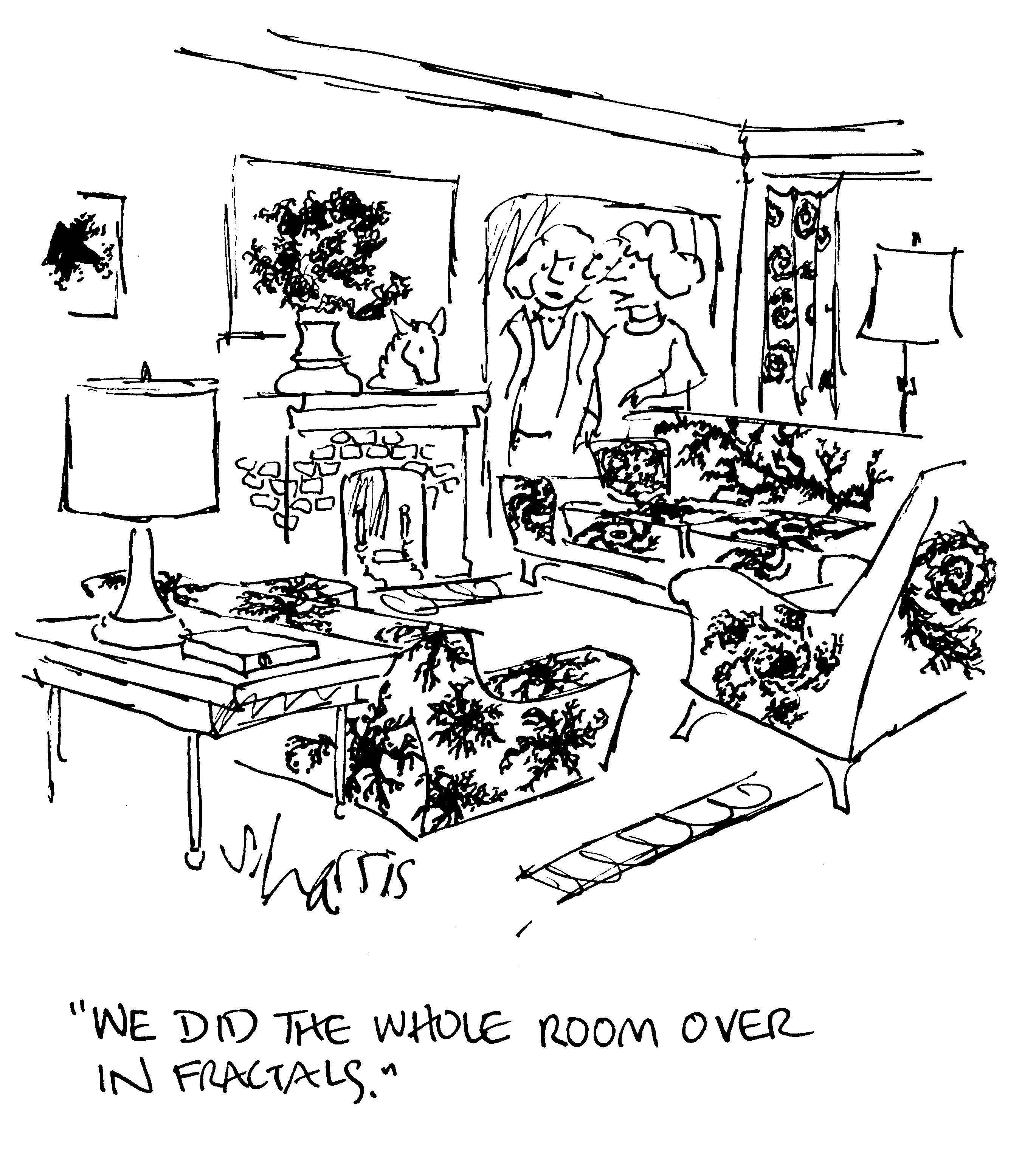# Open Neighborhood Seminar

### Harvard University Math Department

Welcome to Open Neighborhood Seminar! ONS is a general-audience colloquium series for all members of the Harvard math community, including undergraduates at any level. It meets every other Wednesday at 4:30pm in SC507 for an hour-long talk, followed by snacks and a social hour with the speaker. (We alternate with Math Table.) You can subscribe to our mailing list here.

## Next talk: April 19

• Speaker: Curt McMullen (Harvard)
• Title: Illustrating infinity
• Abstract: What do you picture when you think of exp(z), a group of 2x2 matrices, a smooth map, or infinity itself? In this talk we will discuss the practice of mathematical illustration, and give a glimpse of some of the mathematics behind the posters on the first floor of the Science Center.
•## Past and future talks

### February 1

• Speaker: Melanie Matchett Wood (Harvard)
• Title: Universality for groups
• Abstract: The Central Limit Theorem is an example of the ubiquitous yet still surprising phenomena in probability that many random inputs often combine to give an output insensitive to the input distributions. We will explore an example of how this plays out in the construction of random abelian groups from random integral matrices. As an example we will see the probability, as n goes to infinity, that a random linear map from Z^(n+1) to Z^n is surjective. (This talk includes joint work with Hoi Nguyen.)

### February 15

• Speaker: Aaron Landesman (MIT)
• Title: Painleve VI: the search for canonical representations
• Abstract: In 1902, Painleve introduced a collection of 6 differential equations yielding all second order equations with no movable singularities. It turns out that algebraic solutions to Painleve's sixth equation correspond to canonical triples of 2 by 2 complex matrices. We will describe the history of what is known about these canonical tuples of matrices. These canonical tuples can also be viewed as canonical representations of fundamental groups of Riemann surfaces, and also as local systems on certain covers of the moduli space of curves. This talk includes joint work with Daniel Litt.

### March 1

• Speaker: Elden Elmanto (Harvard)
• Title: The joy of negatives
• Abstract: One of the first deep things we learned in elementary school is the act of taking negatives of numbers. I'll explain the geometry that underlies this activity, which leads to algebraic K-theory and other gadgets. I also want to explain the joy of doing math with your friends.

### March 22

• Speaker: Manon Revel (MIT)
• Title: The mathematics of democracy
• Abstract: Is democracy legitimate? It may come as a surprise that mathematicians have contributed to answering this question for a very (very) long time. In this talk, we will explore social choice theory (the maths field researching democratic governance), discuss some of its most striking results, and review the recent developments in understanding how we can make democracy more legitimate. We will look into the probability and random graphs theories underpinning these works, and assess their relevance to the current crises of democracies.

### April 5

• Speaker: Levent Alpoge (Harvard)
• Title: Sums of two cubes
• Abstract: I will connect the following questions and answers.
1. 42 = (12602123297335631)^3 + (80435758145817515)^3 + (-80538738812075974)^3.
2. link, aka: Are there positive integers x, y, z such that: x / (y + z) + y / (x + z) + z / (x + y) = 4?
3. The Birch and Swinnerton-Dyer conjecture.
4. Hilbert's tenth problem, aka: Is there a computer program which "solves all Diophantine equations"?
5. 0% of integers are a sum of two squares (integral or rational).
6. A positive proportion of integers are a sum of two rational cubes.

### April 19

• Speaker: Curt McMullen (Harvard)
• Title: Illustrating infinity
• Abstract: What do you picture when you think of exp(z), a group of 2x2 matrices, a smooth map, or infinity itself? In this talk we will discuss the practice of mathematical illustration, and give a glimpse of some of the mathematics behind the posters on the first floor of the Science Center.
•Organizers: Ana Balibanu (ana@math.harvard.edu) and Dori Bejleri (bejleri@math.harvard.edu). Please drop us an email if you're curious about the seminar!